# PolygonCurve

Polygon >

<Options>

The Polygon command draws a closed polyline with a specified number of sides.#### Steps

• Follow the prompts for the selected option. If no option is specified, the default option is used.
Command-line options

NumSides

Specifies the number of sides for the polygon.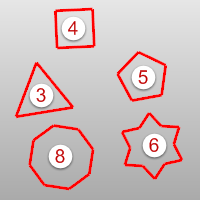Vertical

Draws a polygon perpendicular to the construction plane.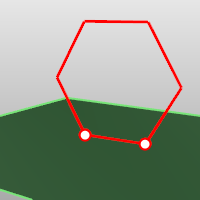AroundCurve

Draws a polygon perpendicular to a curve.#### To create a polygon around a curve

• Select a curve and pick the location on the curve and radius.

Mode

##### Inscribed

Draws the polygon where the corners of the sides touch an imaginary circle of a specified radius.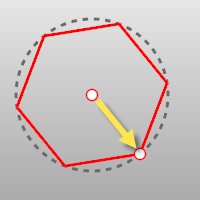#### Inscribed steps

1. Pick the center.
2. Pick a corner.
##### Circumscribed

Draws the polygon where the midpoints of the sides touch an imaginary circle of a specified radius.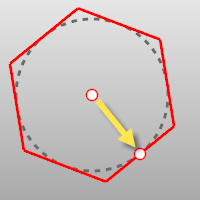#### Circumscribed steps

1. Pick the center.
2. Pick the midpoint of the polygon edge.

Edge

Draws a polygon by defining one edge.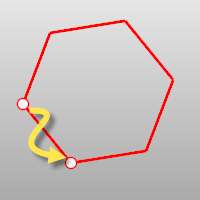#### Edge steps

1. Pick the start of the edge.
2. Pick the end of the edge.
##### Flip

Flips the polygon about the edge you are drawing.Star

Draws a star-shaped polygon.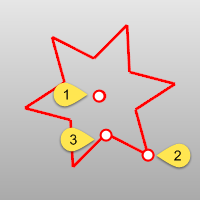#### Star steps

1. Pick the center (1).
2. Pick the corner (2).
3. Pick the radius for the imaginary circle defining the second star radius (3).
##### Automatic

If the polygon has 5 or more sides, the Automatic option creates a star where the size of the outer and inner points are equal.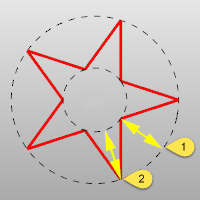Distances 1 and 2 are equal.